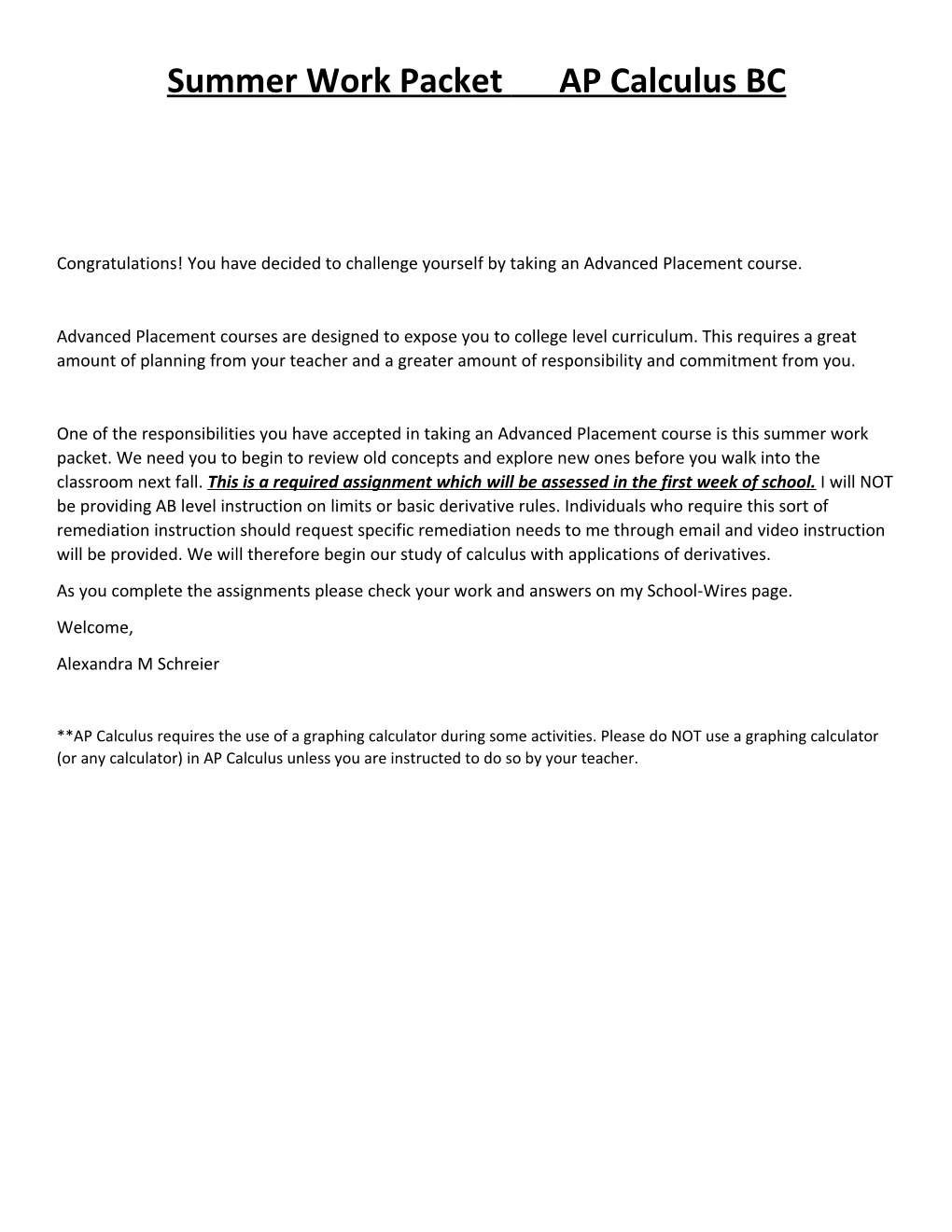# Summer Work Packet AP Calculus BCSummer Work Packet AP Calculus BC

Congratulations! You have decided to challenge yourself by taking an Advanced Placement course.

Advanced Placement courses are designed to expose you to college level curriculum. This requires a great amount of planning from your teacher and a greater amount of responsibility and commitment from you.

One of the responsibilities you have accepted in taking an Advanced Placement course is this summer work packet. We need you to begin to review old concepts and explore new ones before you walk into the classroom next fall. This is a required assignment which will be assessed in the first week of school. I will NOT be providing AB level instruction on limits or basic derivative rules. Individuals who require this sort of remediation instruction should request specific remediation needs to me through email and video instruction will be provided. We will therefore begin our study of calculus with applications of derivatives.

Welcome,

Alexandra M Schreier

**AP Calculus requires the use of a graphing calculator during some activities. Please do NOT use a graphing calculator (or any calculator) in AP Calculus unless you are instructed to do so by your teacher.

AP Style Questions Pre-Calculus

**You may use a graphing calculator to solve the following problems.

1. Let .
1. What is the domain of ?
1. What is the range of ?
1. What are the intercepts of the graph of ?
1. Find .
1. Consider the point and the line .
1. Find the slope of
1. Write an equation for the line through and parallel to
1. Write the equation for the line through and perpendicular to
1. What is the intercept of ?
1. Let
1. What is the domain of ?
1. What is the range of ?
1. What is the period of ?
1. Is an even function, odd function or neither?
1. Find all the zeros of in .

Limits and Continuity

Determine the limit by substitution:

Explain why you cannot use substitution to determine the limit.

Determine the limit using algebra or L’Hospital’s Rule.

Use the graph to estimate the limits and value

of the function, or explain why it does not exist.Determine the limits involving infinity:

AP Style Questions: Limits and Continuity

**You may NOT use a graphing calculator to solve the following problems.

1. Let
1. Find the domain of .
1. Write an equation for each vertical asymptote of the graph of
1. Write an equation for each horizontal asymptote of the graph of .
1. Find all values of for which is discontinuous and classify each discontinuity as removable or non-removable.
1. Let
1. Find .
1. Find .
1. Find all values of that make continuous at . Justify your answer.
1. Let .
1. Find all zeros of .
1. Find a right end behavior model for .
1. Determine and .

Derivatives

Use the definition

1. To find the derivative of the given function at the given value of .
1.Use the graph to the right to

1. Approximate all values of for which

is continuous but not differentiable.

1. Approximate all values of for which

is differentiable but not continuous.

Find :

Find :

Derivatives Part 2

1. Use NDER to find if .
1. Find the equation for the line tangent to the graph of at

.

1. Find the equation of the normal line to the graph of at .
1. Find the value of if the line tangent to the graph of the function at the point passes through the point .
1. What is the slope of the line tangent to the curve

at the point ?

1. At what point on the graph of is the tangent line parallel to the line ?
1. Find an equation for a line that is tangent to the graph of and passes through the origin.

Use the table to answer the questions below:

AP Style Questions: Derivatives

**You may use a graphing calculator to solve the following problems.

1. A particle moves along the axis so that at any time its position is given by .
1. Find the velocity of the particle at any time .
1. Find the acceleration of the particle at any time .
1. Find all values of for which the particle is at rest.
1. Find the speed of the particle when its acceleration is zero.
1. Is the particle moving toward the origin or away from the origin when